Get instant live expert help with Excel or Google Sheets“My Excelchat expert helped me in less than 20 minutes, saving me what would have been 5 hours of work!”

#### Post your problem and you’ll get Expert help in seconds.

Your message must be at least 40 characters
Our professional Expert are available now. Your privacy is guaranteed.

# What to Do if You’re Getting an #N/A Error with VLOOKUP

The VLOOKUP function is very powerful but it can often return the #N/A error message for many different reasons. This article will step you through the troubleshooting process when a VLOOKUP function returns a #N/A error

## Check for Spelling, Capitalization and Extra Spaces

• If either the lookup value or lookup table array is spelled incorrectly, the function will either result in an error or the wrong data being returned. Check to make sure this isn’t the case with your data
• If the lookup table array has capitalized names and the lookup cell (E2) doesn’t or Vise Versa, the formula will return an error because both names have to be completely matching
• There could be an extra space either in the lookup value or lookup table array. This will not allow the formula to function properly. Delete the extra space and your error message should go away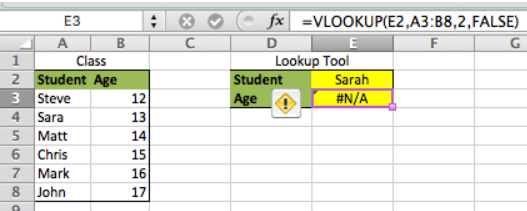Figure 1. #N/A Error with VLOOKUP

In this Example, notice the “h” in Sarah. The lookup cell (E2) spells S-A-R_A_H but the lookup table array spells S-A-R-A which is causing the error. Also, notice the formula how it has “FALSE” for the Approximate Match syntax. When looking up text, you always want to use this concept. Otherwise, there is a risk of pulling in the wrong data and never knowing it. Next, we will use the same example but lookup Mark’s age instead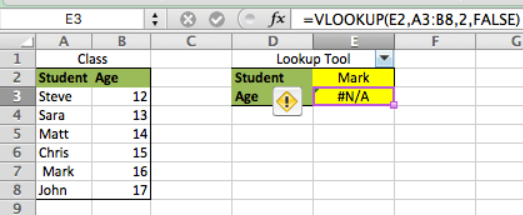Figure 2. #N/A Error with VLOOKUP

In this Example, notice how Mark is spelled correctly in both the lookup cell and the lookup table array and an error message is still showing. Pay special attention to the array table – look at cell A8. There is an extra space in front of Mark’s name. Note, this is obvious here for educational purposes but the extra space is usually less noticeable with regular Excel use. Once we remove the space, the formula will work as intended. Note, the extra space could also be at the end of text which cannot be detected unless you click in the cell. Once we remove the extra spaces, the formula will work as intended

## Data was Deleted or Added

• Sometimes when Rows or Columns are added or deleted to the lookup table array, the VLOOKUP function will return an error because the formula isn’t dynamic enough to consistently react to those changes
• There is no Example of this because of how inconsistent the function is. It works sometimes and then sometimes it doesn’t, so forcing the error can be challenging

## The Lookup Column is not sorted in Ascending Order

• Sometimes when the data in the lookup array isn’t sorted in ascending order, the error message will occur
• Much like when the data is added or deleted, this error is challenging to replicate, so there is not example picture available

## The Lookup Column is not on the Left of the Column to be Returned

• This is one of VLOOKUP’s most undesirable constraints. It is unable to lookup data to the right of the return column
• If we look at our previous example, but instead of attempting to return age, we attempt to lookup any student who’s age is 15, we will not get the result we were looking for. For this Example, we will simply reverse the formula and lookup cells, by placing a VLOOKUP formula in cell E2 and the lookup value in cell E3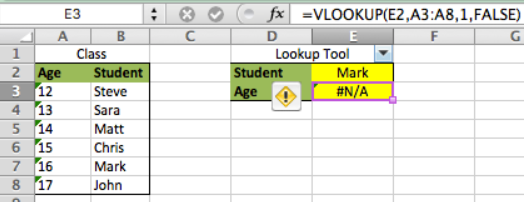Figure 3. #N/A Error with VLOOKUP

We see in this Example that the error message appears because of the data alignment. In order for this concept to work, Columns A and B would have to be reversed with Age being Column A and Student being Column B

## Numbers are Formatted as TEXT

• If you are using an exact match VLOOKUP, then the format for the cells has to be numbers when looking up numeric values or both the lookup table and lookup cell both have to be formatted as TEXT
• We will use the previous example to illustrate this by making the student’s age the lookup value and attempt to return the student’s name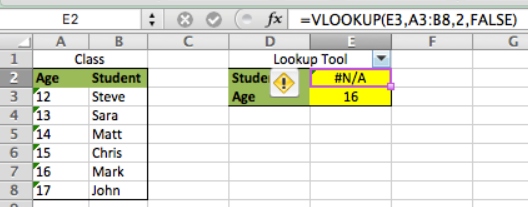Figure 4. #N/A Error with VLOOKUP

Notice how in this Example how the formula returned an error message since the lookup cell was a number and the lookup table array was formatted in TEXT. To correct this, simply change either cell to the other’s format so that they have identical formats

## The Value isn’t Present in the Array

Sometimes the value being looked up isn’t in the lookup array. When this happens, the user will receive the #N/A error message. It can not only be messy, but it can also cause other formulas that depend on the lookup cell to not function properly. Therefore, we must remove that error message without affecting it’s functionality when the lookup value is present in the lookup array. We do this by using the IFERROR function

• The IFERROR function checks for an error where TRUE means there is no error and FALSE means and error is present. Just like the IF function, the user can define what happens in both TRUE and FALSE cases
• IFERROR Syntax = `IFERROR(Action if TRUE,Action if False)`
• We’ll use the previous Example, only we’ll look for a value that isn’t present in the data
• We type this formula in cell E2 `=IFERROR(VLOOKUP(E3,A3:B8,2,FALSE),"")`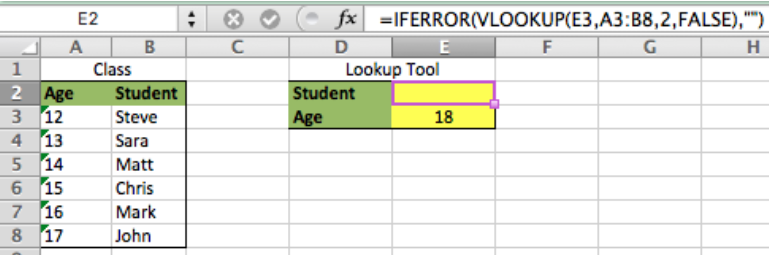Figure 5. #N/A Error with VLOOKUP

We can see in this Example that the formula in cell E2 returned a BLANK value because we told it to in the “Action when FALSE” section of our formula. Now, we are able to make other formulas dependent on cell E2 without seeing an error message.

### Did this post not answer your question? Get a solution from connecting with the expert.Another blog reader asked this question today on Excelchat:
Solution examplesI need to find out if the 2018 yearly sales goals were met if the yearly sales were \$25,000 or more using an IF logical function and to set the formula to return a value of YES if met, and NO if not
Solved by A. A. in 18 minsI filtered data in column A (it is labeled ID #) of a data set. On my next worksheet, column A is also the ID #, but it is a different data set. I want to filter the ID #'s the same for the two sheets, but how do I do that being that I am working with two different sets of data?
Solved by V. H. in 22 minsI'm looking for a formula or format for if there is data in c4 on a sheet... It adds extra information underneath
Solved by X. E. in 20 minshi, my vlookup wont work in some rows but does in others. its temperemental. e.g it will work on rows 10 to 15, then not row 16 and 17, but the will for the rest.
Solved by K. Q. in 21 minsï?·In cell G1enter Discount, ï?·In cell H1enter DiscountPercent,ï?·In cell I1enter DiscountCost,ï?·In cell J1enter TotalCost, andï?·In cell K1enter Paymentas column headings.
Solved by K. E. in 40 mins## Subscribe to Excelchat.coAnother blog reader asked this question today on Excelchat: